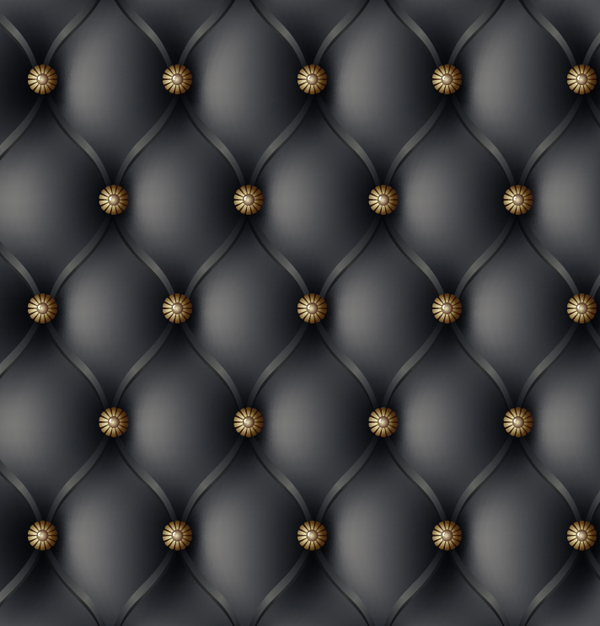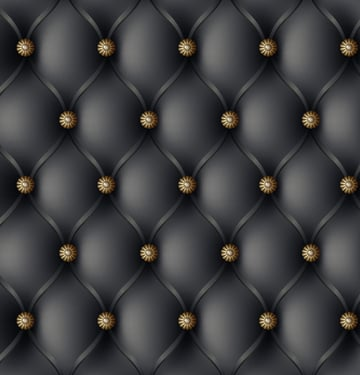# Quick Tip: Create an Upholstery Pattern in Five Minutes!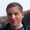In this tutorial you will learn how to create a vector upholstery pattern, using the Gradient Mesh and Transform Effect. Now you can upholster your vector furniture. Let's get started!

## Step 1

Take the Rectangle Tool (M) and create a rectangle 800 by 800 px in size with solid gray fill (C = 51, M = 41, Y = 42 and K = 24).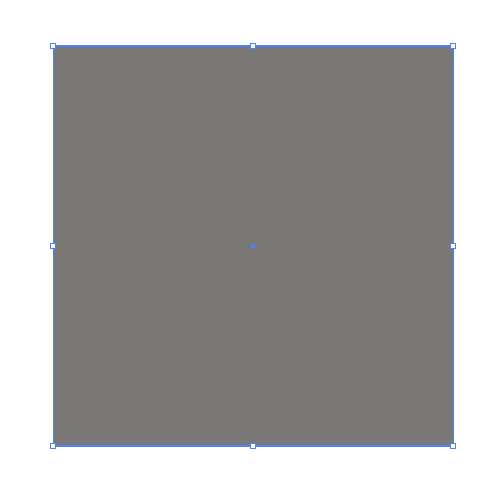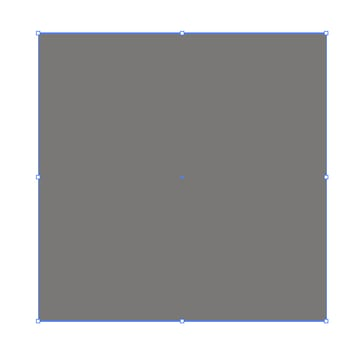Keep the rectangle selected and go to Object > Create Gradient Mesh and put the number of rows and columns in the dialog box. The number of rows must be less than the number of columns so that the grid cell could represent rectangles stretched vertically.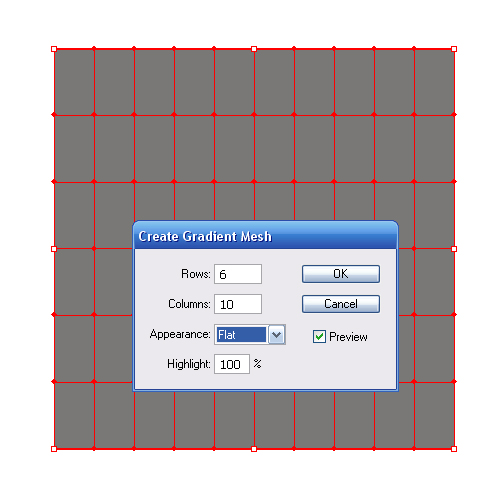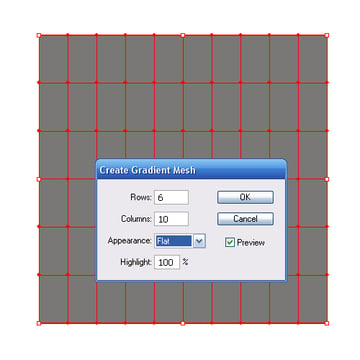## Step 2

Take the Direct Selection Tool (A) and select the gradient mesh nodes checker-wise. Change the color of these points to dark gray (an almost black) color (C = 91, M = 79, Y = 62 and K = 97)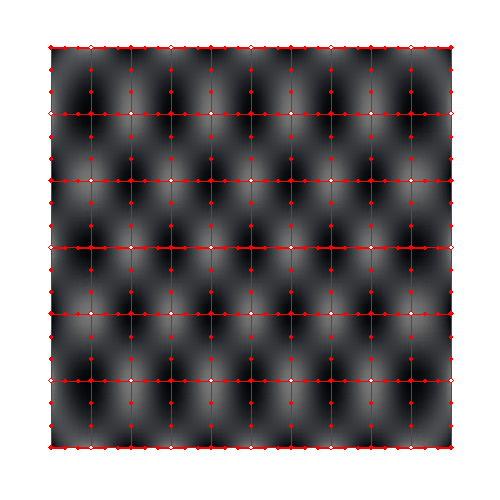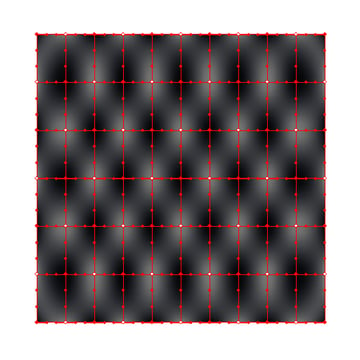## Step 3

Keep these points selected, go to Object > Transform > Move, and set the offset value in the dialog box.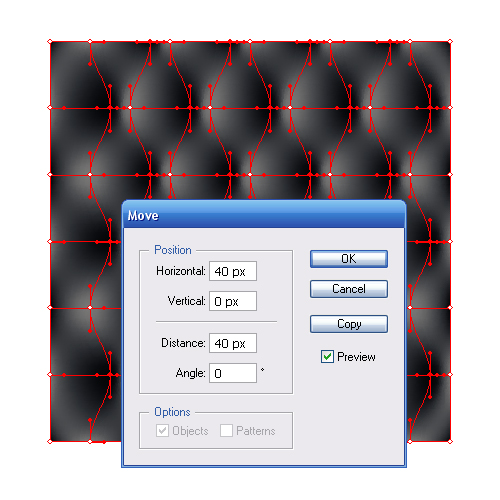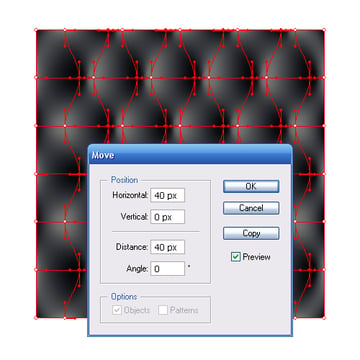## Step 4

Create a ribbon connecting the indents in the skin. Take the Pen Tool (P) and create a line, as shown in the figure below.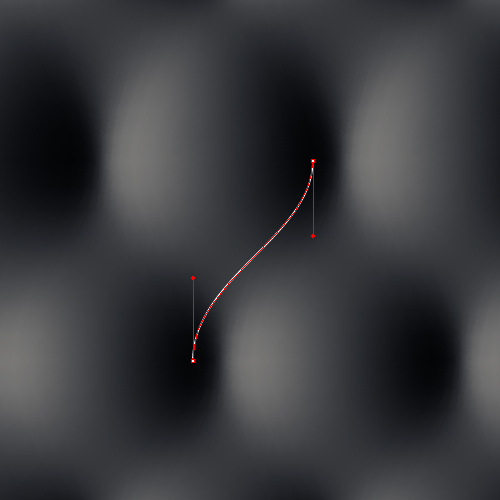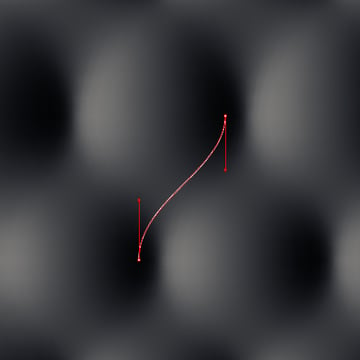Duplicate this line - drag it a little bit to the left while holding down the Option (Alt) Key.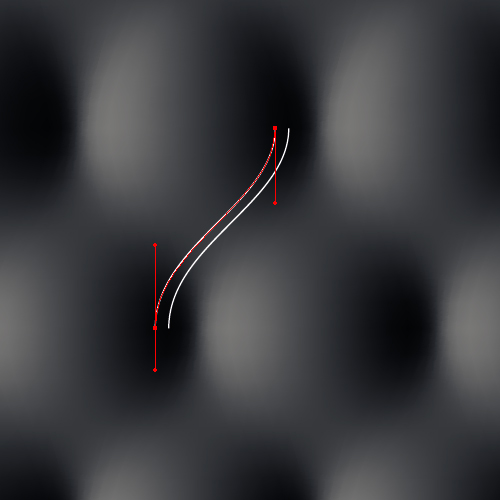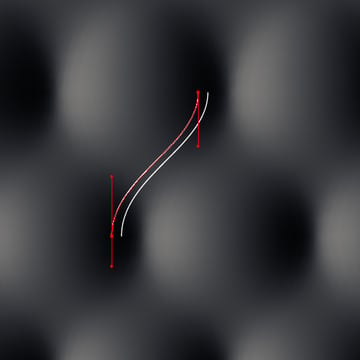Now connect the ends of two lines using the Pen Tool (P).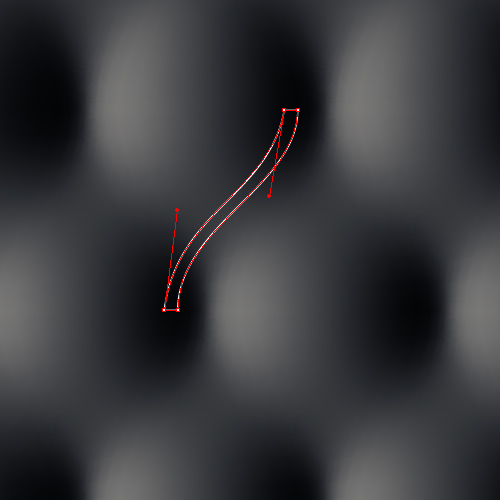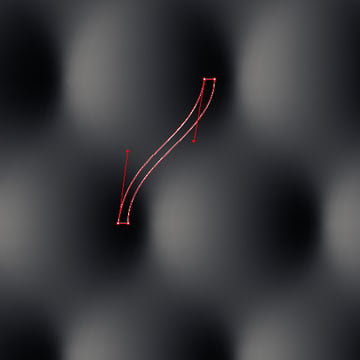Lightly edit the created shape using the Direct Selection Tool (A), and fill it with a linear gradient consisting of the following colors: 1 - (C = 91, M = 79, Y = 62 and K = 97), 2 - (C = 69, M = 59, Y = 58 and K = 68), and 3 - (C = 91, M = 79, Y = 62 and K = 97).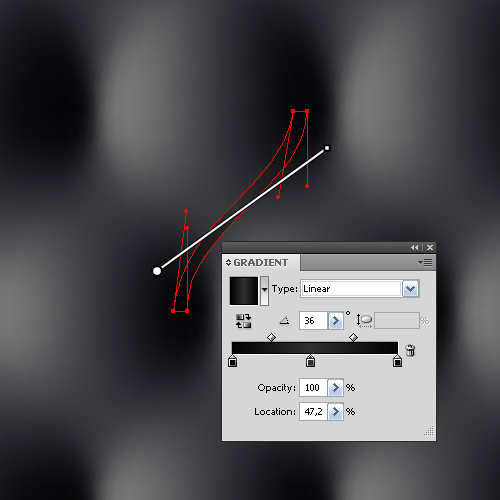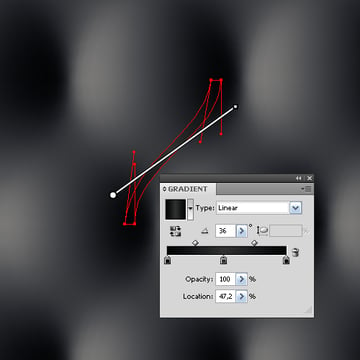## Step 5

Duplicate the created shape moving it slightly to the left. Replace the fill of the copy with a gradient consisting of the following colors: 1 - (C = 91, M = 79, Y = 62 and K = 97), 2 - (C = 57, M = 46, Y = 48 and K = 37) and 3 - (C = 91, M = 79, Y = 62 and K = 97).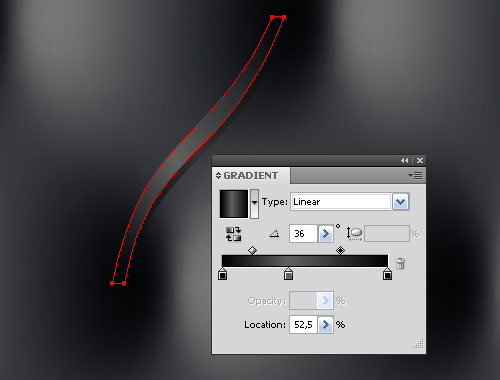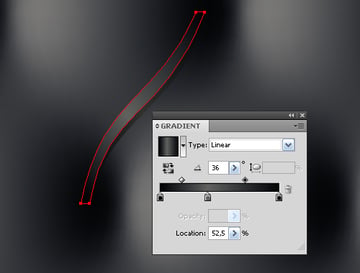## Step 6

Group up both shapes (Command + G) and place the group so that it is located between the nodes of the gradient mesh.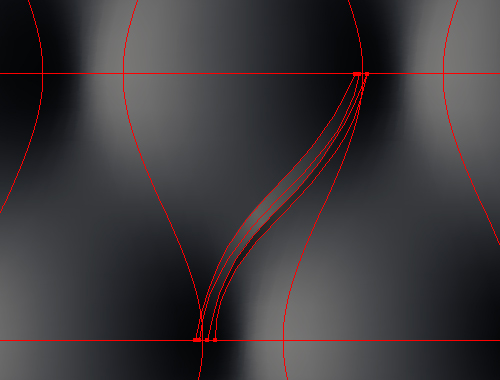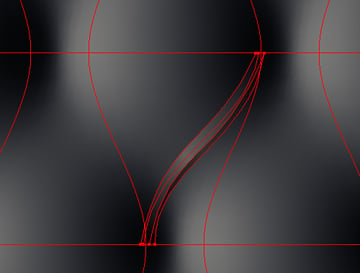Keep the group selected and go to Object > Transform > Reflect and hit the Copy button.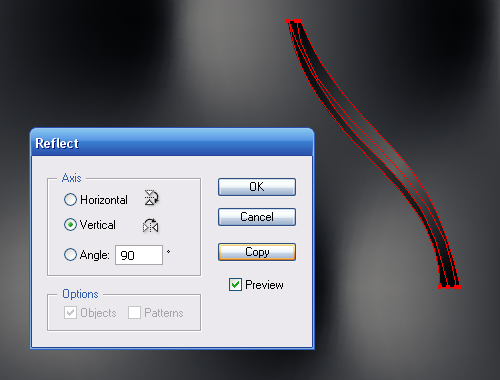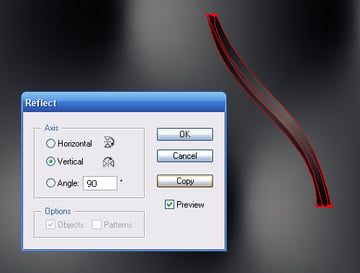Move the copy horizontally to the left holding down the Shift key, in order to form a V, group up these elements.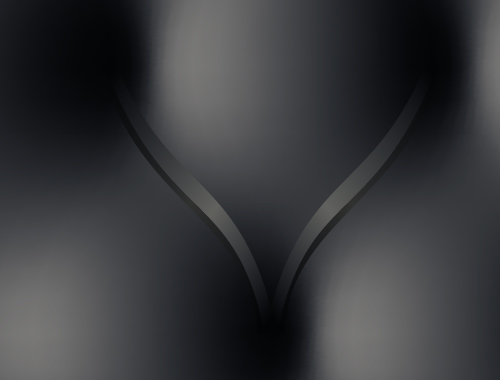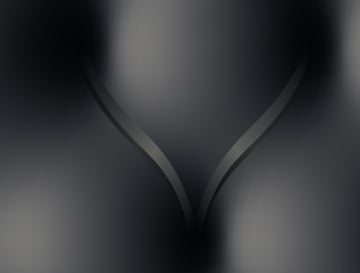## Step 7

Keep the new group selected, go to Effect > Distort & Transform > Transform and set the parameters shown in the figure below.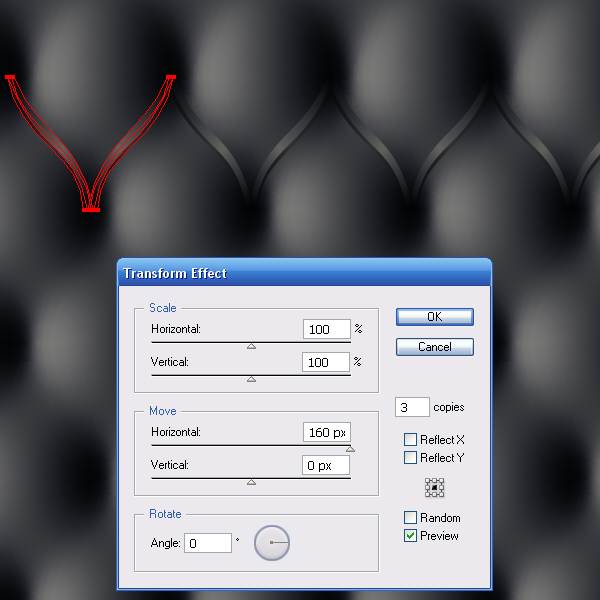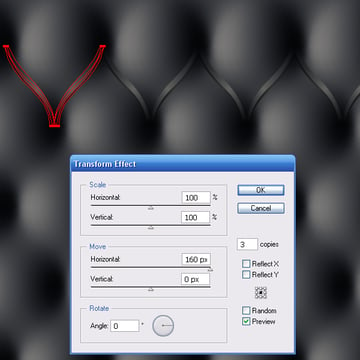Of course, you should understand that the offset value and the number of copies depend on the size of your rectangle and the distance between the node points. Duplicate and place the group with the applied effect over the entire area of the upholstery.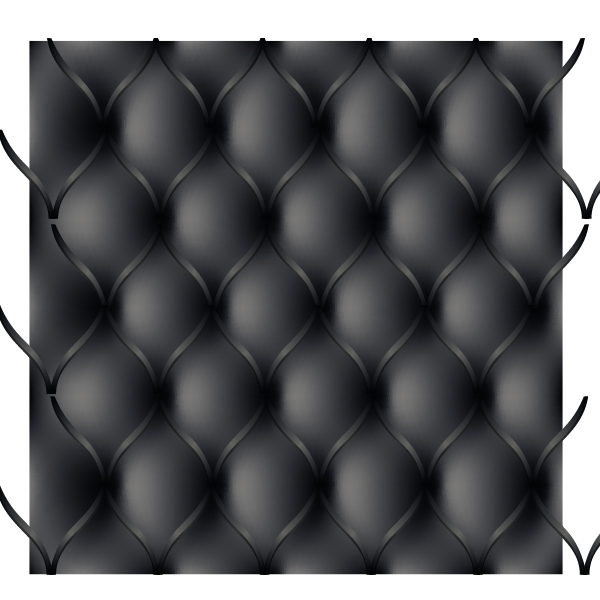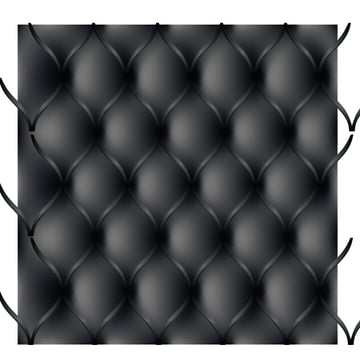You will need to change the number of copies in the transformation effect in some spots. To do this, open the Appearance palette, click on the Transform function and set the number of copies needed in the dialog box.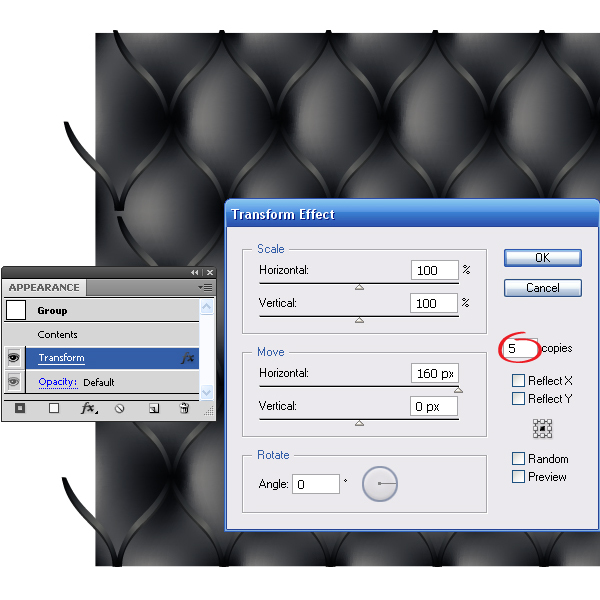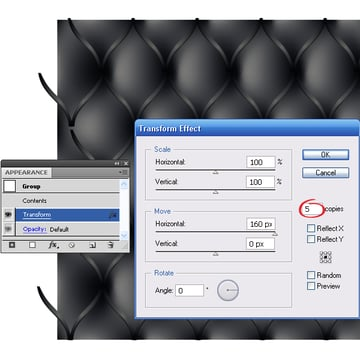## Step 8

Hide the unnecessary pieces of the upholstery. Take the Rectangle Tool (M) and create a rectangle as shown below.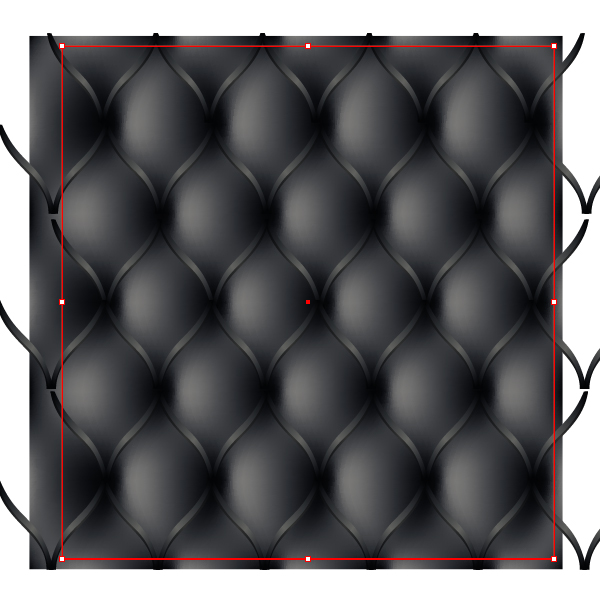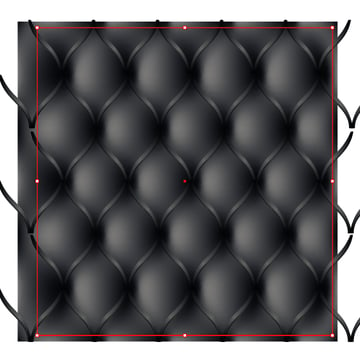The shape you create must be the same as the margins of the upholstery are supposed be. Rectangle will fit for a door, and you can pick an elliptical shape for a chair. Select all the objects (Command + A), and go to Object > Clipping Mask > Make.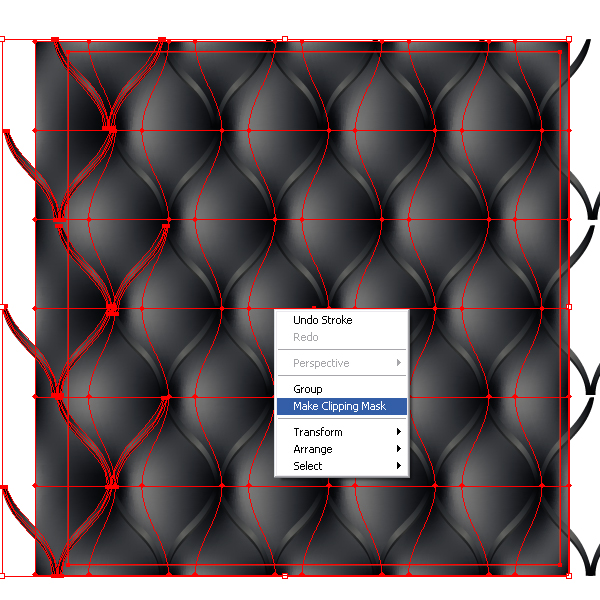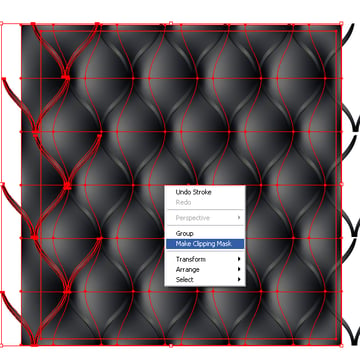## Step 9

Make some ornamental nails, in any style you wish, a simple gradient filled ellipse is enough, or you can make something more ornate, like I have. Place the nails in the indents of the upholstery. Technique of their placement does not differ from the one described in Step 7.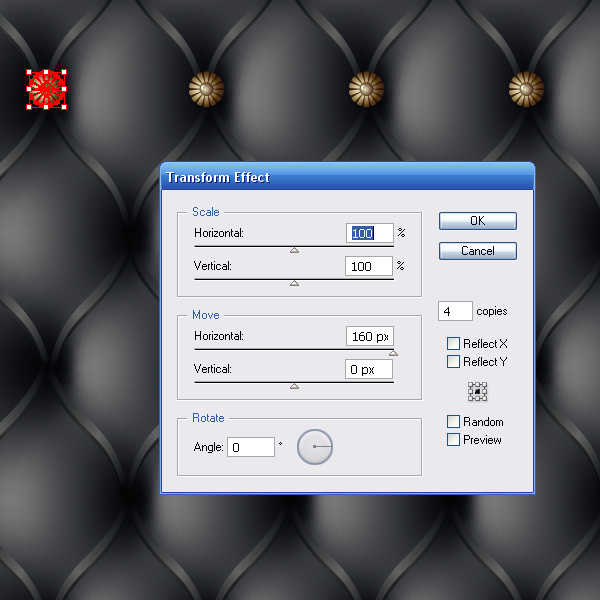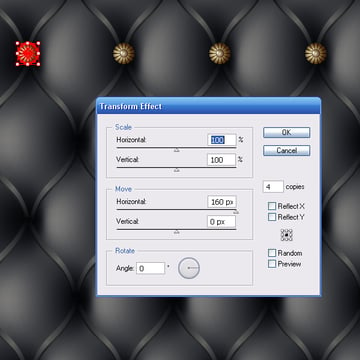## Conclusion

You now have a leather upholstery pattern. If you want to make this pattern a swatch, make a square with no fill and no stroke around an area you wish to repeat. Send the square to the back (Command + Shift + Left Square Bracket) and then select all the objects and drag them into the Swatches Palette. Have fun!